Toll Free: 1-800-676-6123# Simplified Calculations for Choosing Actuator ParametersTemir Baimukhametov
PA Engineer

There are electrical and mechanical considerations that must be taken into account when implementing a linear actuator. It can sometimes be difficult to choose the right size and force. For example, the actuator may be rated to handle twice the weight of a trapdoor in terms of force, but still struggles to open it. This could happen because of the force application angle or insufficient leverage.

In this blog, we will share a set of simplified calculations that would give a good starting point for force and stroke length requirements for a linear actuator.

We have a wide range of electric linear actuators for any application – click to browse!

## Linear Motion

When we talk about linear motion, we mean the movement of the load is linear and this can be in the form of a lift or a pump. This blog will mostly go over vertical motion, but the same techniques could be useful to analyze horizontal motion as well. Let’s take a look at the example in the Figure below.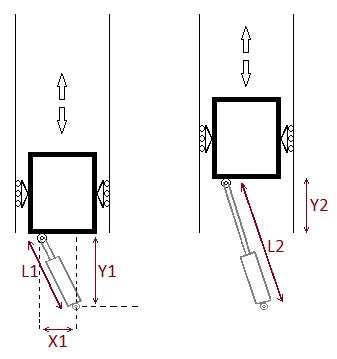Figure 1: Linear motion with Actuator at an angle

 Variable Explanation L1 Length of the actuator when fully retracted. L2 Length of the actuator when fully extended. Y1 Y-axis distance between the front-end mount of the actuator and the rear-end mount. X1 X-axis distance between the front-end mount of the actuator and the read-end mount. Y2 The vertical distance that the load would have to move. S Actuator Stroke – a distance that an actuator extends.

Here we have an actuator at an angle trying to move a mass upwards. The mass has rollers on both sides to indicate that it can only move up and down. The mounting locations on the front and rear end of the actuator are fixed in place so they can only rotate.

## Calculating Stroke Length

If X1was 0, the linear actuator wouldn’t be at an angle anymore so the vertical distance that the load would travel would be equal to the stroke.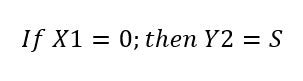Let us examine a more challenging case whereX1 is not 0. Then, to achieve vertical movement of the load, the electric actuator would have to both extend and rotate. This is beneficial because the actuator will take up less space. Then, the stroke length will not be equal to vertical displacement. In order to find the stroke length, we would have to do some light math!

Remember that stroke is simply the difference between the fully extended actuator length and the fully retracted actuator length.The fully retracted and extended actuator lengths are the hypotenuse of a triangle that makes up the rear-end and front-end mounts.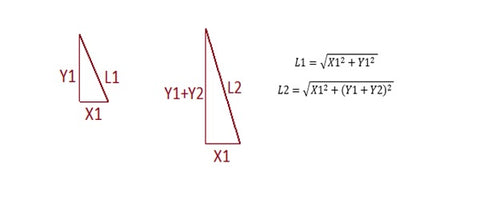Figure 2: Finding Retracted (L1) and Extended (L2) lengths of an actuator

Using this method, we can find the stroke length that would correspond to the desired vertical travel distance of the mass. Based on the formula, the smaller the X1 value is, the more stroke length would correspond to the vertical travel. If X1 is large, small increases in stroke length will lead to large changes in vertical travel distance.

If the actuator was positioned strictly vertically, the change in height will simply be equal to the actuator extension. Positioning the actuator at an angle will increase the total linear movement range of the mass and the actuator could take up less space. That being said, it will also cause some side-loading and we have to be careful not to cause the rod of the actuator to bend. Shorter stroke lengths are recommended when placing the actuator at an angle like this.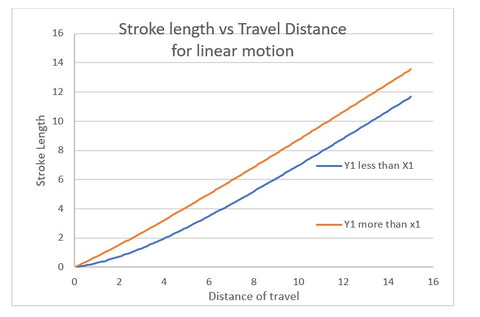Figure 3: Stroke length vs travel distance comparison for linear motion

## Calculating actuator force

We can continue using the property of triangles to find the actuator force. Note that if the actuator is at an angle, the force it is applying will be split into a horizontal and vertical component. The horizontal component of the force doesn’t contribute to the movement. The vertical component of the force will push the mass upwards, so we have to make sure that there is enough force provided by the actuator at all times.Figure 4: Force breakdown for linear motion

We start by calculating the vertical force of the mass.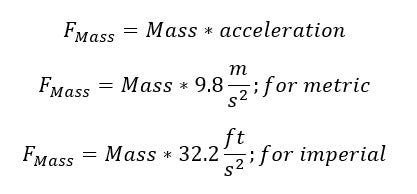We can calculate the required vertical force of the actuator as follows: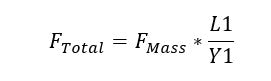Here we are using L1 because the actuator will have the lowest vertical force when fully retracted. When choosing an actuator, we have to make sure that it is capable of dynamic and static force that is higher than F Total that we have calculated.

## Rotary Motion

When we talk about rotary motion, we mean that the load or the mass is rotating around some axis. This could be an application with an opening door or a hatch. It could even be tilting a cargo bed on a truck.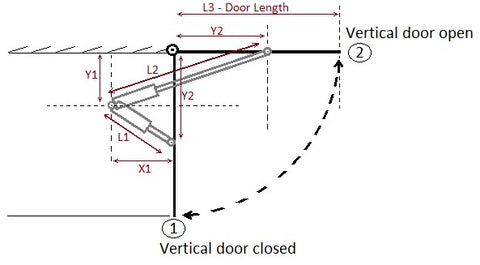Figure 5: Rotary motion with an actuator at an angle

In Figure 5 we are looking at a side view of a vertical door or a hatch that is set up to be opened with a linear actuator. The full retraction of the actuator is indicated by position ① and full extension is indicated by position ②. An actuator is mounted at an angle, both in full extension and in full retraction.

 Variable Explanation L1 Length of the actuator when fully retracted. L2 Length of the actuator when fully extended. Y1 Y-axis distance between the rear-end mount of the actuator and axis of rotation for the door (door hinge). X1 X-axis distance between the rear-end mount of the actuator and axis of rotation for the door (door hinge). Y2 Distance between the door axis of rotation (door hinge) and the front-end mount of the actuator. S Actuator Stroke –  a distance that an actuator extends. L3 The total length of the door.

The example is using the linear actuator mounted at an angle to provide a more general case. If you would like to find the stroke length and force of the actuator when it is mounted perpendicular to the door, you can continue with the guide but set the following: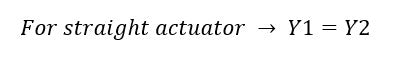## Calculating Stroke Length

We will be using the same triangle method that we used in the linear motion section this time. The only change is the triangles are constructed differently this time.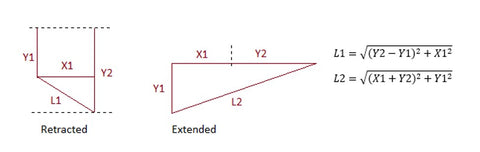Figure 6: Finding retracted (L1) and extended (L2) actuator length

Same as before, the stroke length is the difference between the fully extended and fully retracted length of the actuator. We can find it simply as follows:In this case, the actuator stroke length heavily depends on the location of the front-end and rear-end mounts. The closer we place the front-end mount to the hinge of the door, the less the actuator will have to travel to open or close the door. Similarly, the closer the rear-end mount is to the hinge, the less stroke is required to open the door.

There is an inflection point where moving the actuator further away from the hinge no longer causes large changes in actuator stroke length because the actuator length closely matches the door length and most of the movement is done through the rotation. This is not a good position for the actuator because the leverage is very poor, but we will discuss that in the further sections.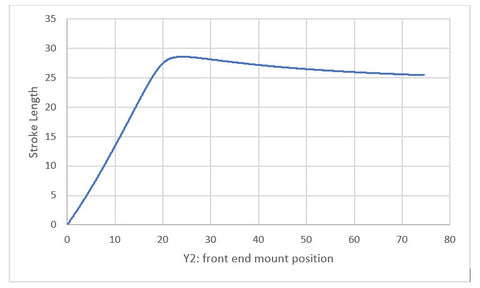Figure 7: Stroke length relative to front-end mount position (aka, distance from front end mount to door hinge)Figure 8: Stroke length relative to the rear-end mount position

We can see from Figure 8 that varying rear-end mount position does affect the stroke length required, but the effect tends to level-off quite quickly.

## Calculating Actuator Force

In order to find the force rating for our actuator, we will need to determine the expected load of the door. Because the door is rotating around the door hinges, simply knowing the mass of the door is not enough to determine the force exerted on the actuator. For this application, we will need to find the mass moment of inertia of the door.

Intuitively, we know that opening a door using a doorknob (located far away from the hinge) is much easier than opening a door by pushing somewhere near the hinge.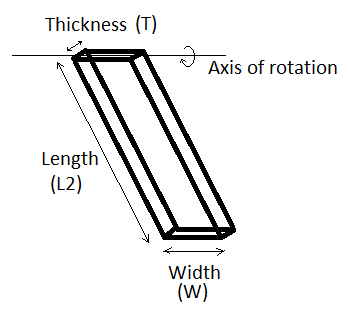Figure 9: Representation of a door opening vertically

The moment of inertia (denoted as I) for the vertically opening door around the hinge can be found as follows:Now that we have the moment of inertia, we know the torque that the actuator has to apply to the door to make it move. Therefore, we can calculate the force as follows: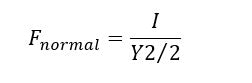This force is called F normal because this is just one component of the force applied to the actuator and not the full force. It is better illustrated in Figure 10. As you can see, F normal is not acting along L1 or L2 line, but at an angle.Figure 10: Mounting location of the actuators

This means we have to convert F normal from just a component force to full actuator force. Because our load is a rotating door, the F normal force stays constant, but the load applied to the actuator changes. For example, when the actuator is fully retracted in position ①, the door hinge is taking most of the load, so the actuator won’t experience much force until it has to move the door. On the other hand, when the actuator is in full extension in position ② the door hinge is not supporting the door as much. In that case, the actuator has to hold the bulk of the load.

We can calculate the retracted and the extended force needed for the actuator. Depending on the mounting conditions, the force in the extended position could be higher than the force in the retracted position or it can be the reverse. For this reason, we have to calculate both and choose the highest to make sure our application is robust.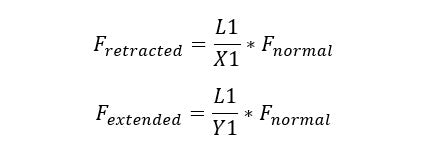For example, in Figure 10, the highest force would be applied to the actuator when it is fully extended. Then, the minimum force rating for the actuator would have to be equal to or higher than F extended.

## Conclusion

In this blog, we looked at simplified ways of calculating the desired force rating and stroke length for linear actuators. The equations in this blog can be used to calculate approximate requirements for the linear and rotary motion of the load. Reach out to us at sales@progressiveautomations.com for any further questions and our team of engineers would be happy to assist you.